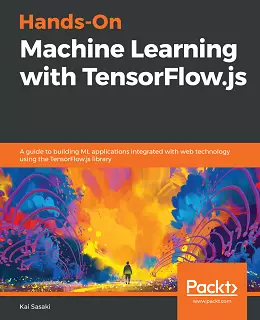# Hands-On Machine Learning with TensorFlow.js### eBook Details:

• Paperback: 296 pages
• Publisher: WOW! eBook (November 27, 2019)
• Language: English
• ISBN-10: 1838821732
• ISBN-13: 978-1838821739

### eBook Description:

Hands-On Machine Learning with TensorFlow.js: Your one-stop guide to working with the browser-based JavaScript library for training and deploying machine learning models effectively

TensorFlow.js is a framework that enables you to create performant machine learning (ML) applications that run smoothly in a web browser. With this book, you will learn how to use TensorFlow.js to implement various ML models through an example-based approach.

Starting with the basics, you’ll understand how ML models can be built on the web. Moving on, you will get to grips with the TensorFlow.js ecosystem to develop applications more efficiently. The book will then guide you through implementing ML techniques and algorithms such as regression, clustering, fast Fourier transform (FFT), and dimensionality reduction. You will later cover the Bellman equation to solve Markov decision process (MDP) problems and understand how it is related to reinforcement learning. Finally, you will explore techniques for deploying ML-based web applications and training models with TensorFlow Core. Throughout this ML book, you’ll discover useful tips and tricks that will build on your knowledge.

• Use the t-SNE algorithm in TensorFlow.js to reduce dimensions in an input dataset
• Deploy tfjs-converter to convert Keras models and load them into TensorFlow.js
• Apply the Bellman equation to solve MDP problems
• Use the k-means algorithm in TensorFlow.js to visualize prediction results
• Create tf.js packages with Parcel, Webpack, and Rollup to deploy web apps
• Implement tf.js backend frameworks to tune and accelerate app performance

By the end of this Hands-On Machine Learning with TensorFlow.js book, you will be equipped with the skills you need to create your own web-based ML applications and fine-tune models to achieve high performance.

### 1 Response

1. April 3, 2020

[…] giving you an overview of what’s new in TensorFlow 2.0 Alpha, the book moves on to setting up your machine learning environment using the TensorFlow library. […]